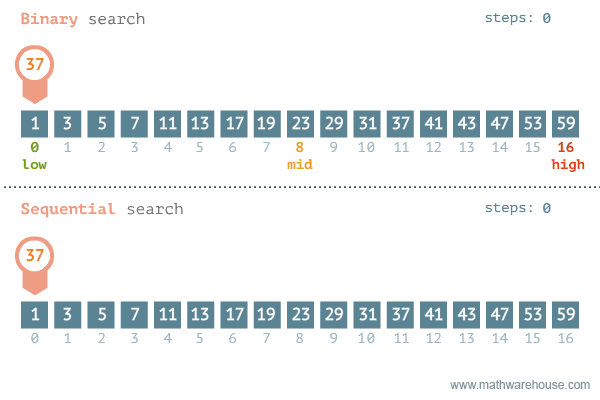2022-09-1600

# 面试官：说说你对二分查找的理解？如何实现？应用场景？## 一、是什么

• 存储在数组中
• 有序排序## 二、如何实现

``````function BinarySearch(arr, target) {
if (arr.length <= 1) return -1
// 低位下标
let lowIndex = 0
// 高位下标
let highIndex = arr.length - 1

while (lowIndex <= highIndex) {
// 中间下标
const midIndex = Math.floor((lowIndex + highIndex) / 2)
if (target < arr[midIndex]) {
highIndex = midIndex - 1
} else if (target > arr[midIndex]) {
lowIndex = midIndex + 1
} else {
// target === arr[midIndex]
return midIndex
}
}
return -1
}``````

``````function BinarySearchFirst(arr, target) {
if (arr.length <= 1) return -1
// 低位下标
let lowIndex = 0
// 高位下标
let highIndex = arr.length - 1

while (lowIndex <= highIndex) {
// 中间下标
const midIndex = Math.floor((lowIndex + highIndex) / 2)
if (target < arr[midIndex]) {
highIndex = midIndex - 1
} else if (target > arr[midIndex]) {
lowIndex = midIndex + 1
} else {
// 当 target 与 arr[midIndex] 相等的时候，如果 midIndex 为0或者前一个数比 target 小那么就找到了第一个等于给定值的元素，直接返回
if (midIndex === 0 || arr[midIndex - 1] < target) return midIndex
// 否则高位下标为中间下标减1，继续查找
highIndex = midIndex - 1
}
}
return -1
}``````• 分界点元素 >= 第一个元素
• 分界点元素 < 第一个元素

``````function search (nums, target) {
// 如果为空或者是空数组的情况
if (nums == null || !nums.length) {
return -1;
}
// 搜索区间是前闭后闭
let begin = 0,
end = nums.length - 1;
while (begin <= end) {
// 下面这样写是考虑大数情况下避免溢出
let mid = begin + ((end - begin) >> 1);
if (nums[mid] == target) {
return mid;
}
// 如果左边是有序的
if (nums[begin] <= nums[mid]) {
//同时target在[ nums[begin],nums[mid] ]中，那么就在这段有序区间查找
if (nums[begin] <= target && target <= nums[mid]) {
end = mid - 1;
} else {
//否则去反方向查找
begin = mid + 1;
}
//如果右侧是有序的
} else {
//同时target在[ nums[mid],nums[end] ]中，那么就在这段有序区间查找
if (nums[mid] <= target && target <= nums[end]) {
begin = mid + 1;
} else {
end = mid - 1;
}
}
}
return -1;
};``````

## 三、应用场景

• 有序：我们很难保证我们的数组都是有序的
• 数组：数组读取效率是O(1)，可是它的插入和删除某个元素的效率却是O(n)，并且数组的存储是需要连续的内存空间，不适合大数据的情况

• 不适合数据量太小的数列；数列太小，直接顺序遍历说不定更快，也更简单
• 每次元素与元素的比较是比较耗时的，这个比较操作耗时占整个遍历算法时间的大部分，那么使用二分查找就能有效减少元素比较的次数
• 不适合数据量太大的数列，二分查找作用的数据结构是顺序表，也就是数组，数组是需要连续的内存空间的，系统并不一定有这么大的连续内存空间可以使用

## 参考文献